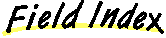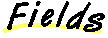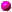`All Packages  Class Hierarchy  This Package  Previous  Next  Index`

# Class java.awt.Polygon

```java.lang.Object
|
+----java.awt.Polygon
```

public class Polygon
extends Object
implements Shape, Serializable
A polygon consists of a list of x and y coordinates.

##boundsnpoints
The total number of points.xpoints
The array of x coordinates.ypoints
The array of y coordinates.

##Polygon()
Creates an empty polygon.Polygon(int[], int[], int)
Constructs and initializes a Polygon from the specified parameters.

##addPoint(int, int)
Appends a point to a polygon.contains(int, int)
Determines whether the point (x,y) is inside the Polygon.contains(Point)
Determines whether the specified point is inside the Polygon.getBoundingBox()
Deprecated.getBounds()
Returns the bounding box of the shape.inside(int, int)
Deprecated.translate(int, int)
Translates the vertices by deltaX, deltaY.

##npoints
``` public int npoints
```
The total number of points.xpoints
``` public int xpoints[]
```
The array of x coordinates.ypoints
``` public int ypoints[]
```
The array of y coordinates.bounds
``` protected Rectangle bounds
```

##Polygon
``` public Polygon()
```
Creates an empty polygon.Polygon
``` public Polygon(int xpoints[],
int ypoints[],
int npoints)
```
Constructs and initializes a Polygon from the specified parameters.

Parameters:
xpoints - the array of x coordinates
ypoints - the array of y coordinates
npoints - the total number of points in the Polygon

##translate
``` public void translate(int deltaX,
int deltaY)
```
Translates the vertices by deltaX, deltaY.

Parameters:
deltaX - the amount to move the X coords
deltaY - the amount to move the Y coordsaddPoint
``` public void addPoint(int x,
int y)
```
Appends a point to a polygon. If inside(x, y) or another operation that calculates the bounding box has already been performed, this method updates the bounds accordingly.

Parameters:
x - the x coordinate of the point
y - the y coordinate of the pointgetBounds
``` public Rectangle getBounds()
```
Returns the bounding box of the shape.

Returns:
a Rectangle defining the bounds of the Polygon.getBoundingBox
``` public Rectangle getBoundingBox()
```
Note: getBoundingBox() is deprecated. As of JDK version 1.1, replaced by getBounds().contains
``` public boolean contains(Point p)
```
Determines whether the specified point is inside the Polygon. Uses an even-odd insideness rule (also known as an alternating rule).

Parameters:
p - the point to be testedcontains
``` public boolean contains(int x,
int y)
```
Determines whether the point (x,y) is inside the Polygon. Uses an even-odd insideness rule (also known as an alternating rule).

Parameters:
x - the X coordinate of the point to be tested
y - the Y coordinate of the point to be tested

Kindly donated by Hanpeter van Vliet .inside
``` public boolean inside(int x,
int y)
```
Note: inside() is deprecated. As of JDK version 1.1, replaced by contains(int, int).

`All Packages  Class Hierarchy  This Package  Previous  Next  Index`

Submit a bug or feature